# SAS Macros and Functions

### PROBDF Function for Dickey-Fuller Tests

Subsections:

The PROBDF function calculates significance probabilities for Dickey-Fuller tests for unit roots in time series. The PROBDF function can be used wherever SAS library functions can be used, including DATA step programs, SCL programs, and PROC MODEL programs.

#### Syntax

PROBDF( x, n < , d < , type > > )

x

is the test statistic.

n

is the sample size. The minimum value of n allowed depends on the value specified for the third argument d. For d in the set (1,2,4,6,12), n must be an integer greater than or equal to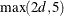; for other values of d the minimum value of n is 24.

d

is an optional integer giving the degree of the unit root tested for. Specifyfor tests of a simple unit root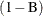. Specify d equal to the seasonal cycle length for tests for a seasonal unit root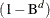. The default value of d is 1; that is, a test for a simple unit rootis assumed if d is not specified. The maximum value of d allowed is 12.

type

is an optional character argument that specifies the type of test statistic used. The values of type are the following:

SZM

studentized test statistic for the zero mean (no intercept) case

RZM

regression test statistic for the zero mean (no intercept) case

SSM

studentized test statistic for the single mean (intercept) case

RSM

regression test statistic for the single mean (intercept) case

STR

studentized test statistic for the deterministic time trend case

RTR

regression test statistic for the deterministic time trend case

The values STR and RTR are allowed only when. The default value of type is SZM.

#### Details

##### Theoretical Background

When a time series has a unit root, the series is nonstationary and the ordinary least squares (OLS) estimator is not normally distributed. The limiting distribution of the OLS estimator of autoregressive models for time series with a simple unit root was studied by Dickey (1976); Dickey and Fuller (1979). Dickey, Hasza, and Fuller (1984) obtained the limiting distribution for time series with seasonal unit roots. We will mainly introduce the nonseasonal tests in the following and list references for the nonseasonal tests.

Consider the Dickey-Fuller regression first. The null hypothesis is that there is an autoregressive unit root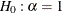, and the alternative is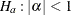, where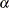is the autoregressive coefficient of the time series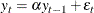This is referred to as the zero mean (ZM) model. The standard Dickey-Fuller (DF) test assumes that errors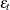are white noise. There are two other types of regression models that include a constant or a time trend as follows: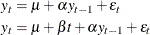These two models are referred to as the constant mean model (SM) and the trend model (TR), respectively. The constant mean model includes a constant meanof the time series. However, the interpretation ofdepends on the stationarity in the following sense: the mean in the stationary case when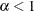is the trend in the integrated case when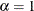. Therefore, the null hypothesis should be the joint hypothesis thatand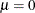. However for the unit root tests, the test statistics are concerned with the null hypothesis of. The joint null hypothesis is not commonly used. This issue is address in Bhargava (1986) with a different nesting model.

Under the null of I(1) of the Dickey-Fuller test, the differenced process is not serially correlated. There is a great need for the generalization of this specification. The augmented Dickey-Fuller (ADF) test, originally proposed in Dickey and Fuller (1979), adjusts for the serial correlation in the time series by adding lagged first differences to the autoregressive model as follows. Consider the (p +1)th order autoregressive time series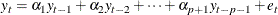and its characteristic equation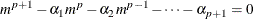If all the characteristic roots are less than 1 in absolute value,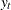is stationary.is nonstationary if there is a unit root. If there is a unit root, the sum of the autoregressive parameters is 1, and hence you can test for a unit root by testing whether the sum of the autoregressive parameters is 1 or not. The no-intercept model is parameterized as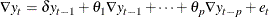where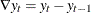and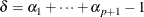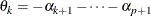The estimators are obtained by regressing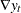on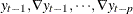. The t statistic of the ordinary least squares estimator of
is the test statistic for the unit root test.

If the type argument value specifies a test for a nonzero mean (intercept case), the autoregressive model includes a mean term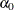. If the type argument value specifies a test for a time trend, the model also includes a time trend term and the model is as follows: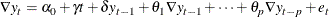For testing for a seasonal unit root, consider the multiplicative model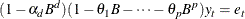Let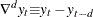. The test statistic is calculated in the following steps:

1. Regress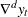on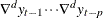to obtain the initial estimators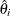and compute residuals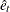. Under the null hypothesis that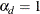,are consistent estimators of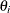.

2. Regresson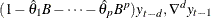,,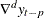to obtain estimates of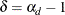and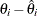.

The t ratio for the estimate ofproduced by the second step is used as a test statistic for testing for a seasonal unit root. The estimates ofare obtained by adding the estimates offrom the second step tofrom the first step.

The series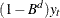is assumed to be stationary, where d is the value of the third argument to the PROBDF function.

If the series is an ARMA process, a large value of p might be desirable in order to obtain a reliable test statistic. To determine an appropriate value for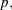see Said and Dickey (1984).

##### Test Statistics

The Dickey-Fuller test is used to test the null hypothesis that the time series exhibits a lag d unit root against the alternative of stationarity. The PROBDF function computes the probability of observing a test statistic more extreme than x under the assumption that the null hypothesis is true. You should reject the unit root hypothesis when PROBDF returns a small (significant) probability value.

Consider the Dickey-Fuller regression first. There are several different versions of the Dickey-Fuller test. The PROBDF function supports six versions, as selected by the type argument. Specify the type value that corresponds to the way that you calculated the test statistic x.

The last two characters of the type value specify the kind of regression model used to compute the Dickey-Fuller test statistic. The meaning of the last two characters of the type value are as follows:

ZM

zero mean or no-intercept case. The test statistic x is assumed to be computed from the regression model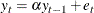SM

single mean or intercept case. The test statistic x is assumed to be computed from the regression model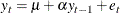TR

intercept and deterministic time trend case. The test statistic x is assumed to be computed from the regression model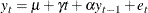The first character of the type value specifies whether the regression test statistic or the studentized test statistic is used. Let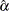be the estimated regression coefficient for the lag of the series, and let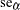be the standard error of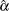. The meaning of the first character of the type value is as follows:

R

the regression-coefficient-based test statistic. The test statistic is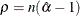S

the studentized test statistic. The test statistic is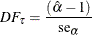The first one is also called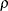-test and the second is called-test. For the zero mean model, the asymptotic distributions of the Dickey-Fuller test statistics are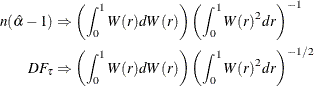For the constant mean model, the asymptotic distributions are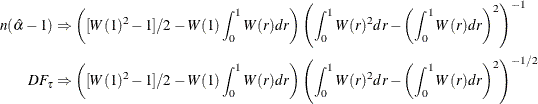For the trend model, the asymptotic distributions are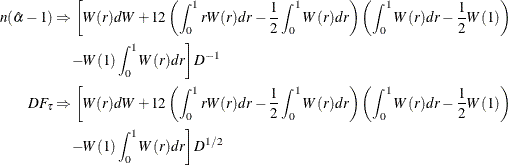where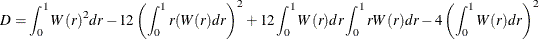For more information about the Dickey-Fuller test null distribution see: Dickey and Fuller (1979); Dickey, Hasza, and Fuller (1984); Hamilton (1994). The preceding formulas are for the basic Dickey-Fuller test. The PROBDF function can also be used for the augmented Dickey-Fuller test, in which the error term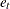is modeled as an autoregressive process; however, the test statistic is computed somewhat differently for the augmented Dickey-Fuller test. For the nonseasonal augmented Dickey-Fuller test, the test statistics can take one of the two forms similar to Dickey-Fuller test. One is the OLS t value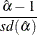and the other is given by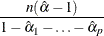The asymptotic distributions of the test statistics are the same as those of the standard Dickey-Fuller test statistics. For information about seasonal and nonseasonal augmented Dickey-Fuller tests see: Dickey, Hasza, and Fuller (1984); Hamilton (1994).

The PROBDF function is calculated from approximating functions fit to empirical quantiles that are produced by a Monte Carlo simulation that employs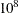replications for each simulation. Separate simulations were performed for selected values of n and for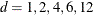(where n and d are the second and third arguments to the PROBDF function).

The maximum error of the PROBDF function is approximately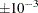for d in the set (1,2,4,6,12) and can be slightly larger for other d values. Because the number of simulation replications used to produce the PROBDF function is much greater than the 60,000 replications used by Dickey and colleagues (Dickey and Fuller, 1979; Dickey, Hasza, and Fuller, 1984), the PROBDF function can be expected to produce results that are substantially more accurate than the critical values reported in those papers.

#### Examples

Suppose the data set TEST contains 104 observations of the time series variable Y, and you want to test the null hypothesis that there exists a lag 4 seasonal unit root in the Y series. The following statements illustrate how to perform the single-mean Dickey-Fuller regression coefficient test using PROC REG and PROBDF.

   data test1;
set test;
y4 = lag4(y);
run;

proc reg data=test1 outest=alpha;
model y = y4 / noprint;
run;

data _null_;
set alpha;
x = 100 * ( y4 - 1 );
p = probdf( x, 100, 4, "RSM" );
put p= pvalue5.3;
run;


To perform the augmented Dickey-Fuller test, regress the differences of the series on lagged differences and on the lagged value of the series, and compute the test statistic from the regression coefficient for the lagged series. The following statements illustrate how to perform the single-mean augmented Dickey-Fuller studentized test for a simple unit root using PROC REG and PROBDF:

   data test1;
set test;
yl  = lag(y);
yd  = dif(y);
yd1 = lag1(yd); yd2 = lag2(yd);
yd3 = lag3(yd); yd4 = lag4(yd);
run;

proc reg data=test1 outest=alpha covout;
model yd = yl yd1-yd4 / noprint;
run;

data _null_;
set alpha;
retain a;
if _type_ = 'PARMS' then a = yl ;
if _type_ = 'COV' & _NAME_ = 'Y1' then do;
x = a / sqrt(yl);
p = probdf( x, 99, 1, "SSM" );
put p= pvalue5.3;
end;
run;


The %DFTEST macro provides an easier way to perform Dickey-Fuller tests. The following statements perform the same tests as the preceding example:

   %dftest( test, y, ar=4 );
%put p=&dftest;